#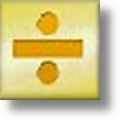Division Worksheets

## Printable Division Worksheets for Teachers

Here is a graphic preview for all of the division worksheets. You can select different variables to customize these division worksheets for your needs. The division worksheets are randomly created and will never repeat so you have an endless supply of quality division worksheets to use in the classroom or at home. Our division worksheets are free to download, easy to use, and very flexible.

## Quick Link for All Division Worksheets

Click the image to be taken to that Division Worksheet.

##### Divisibility Rules HandoutDivision Worksheets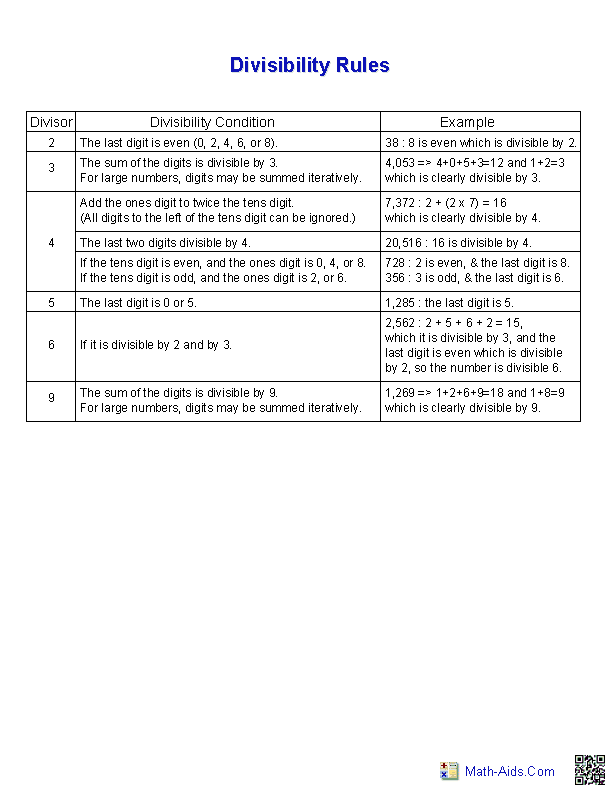##### Divisibility Test ProblemsDivision Worksheets##### Divisibility - Pick DivisorsDivision Worksheets##### Single or Multi DigitDivision Worksheets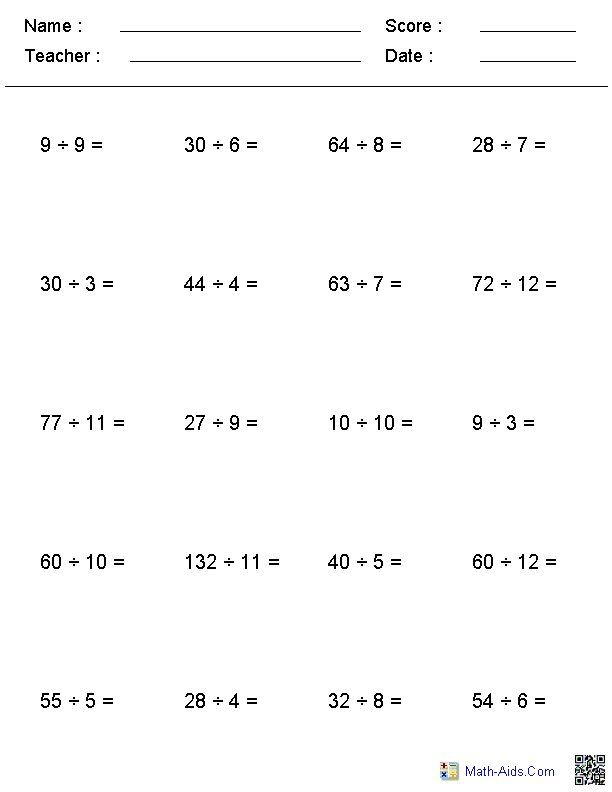##### Long DivisionDivision Worksheets##### Short Division - 1 DivisorDivision Worksheets##### Decimal LongDivision Worksheets##### Decimal DivisorDivision Worksheets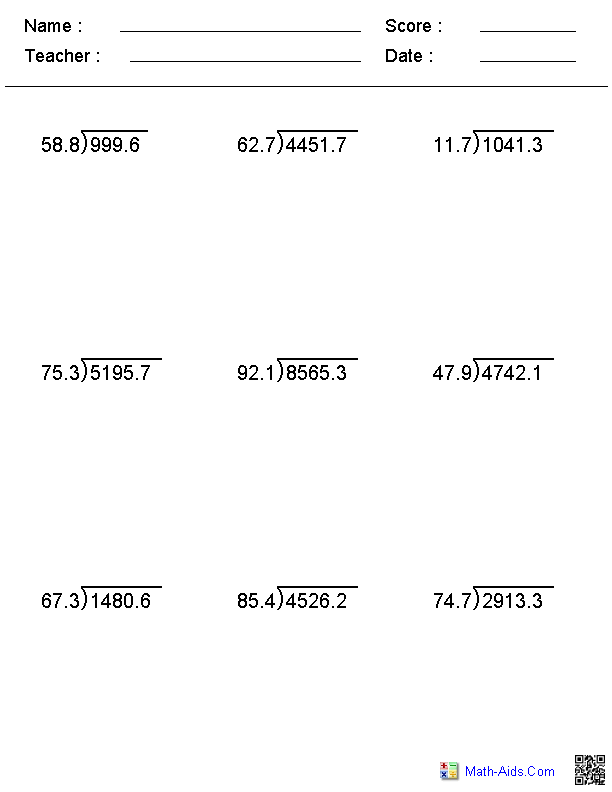##### 3 Digit DecimalDivision Worksheets##### Mixed Quotient DivisionDivision Worksheets##### 1, 3, or 5 Minute DrillsDivision Worksheets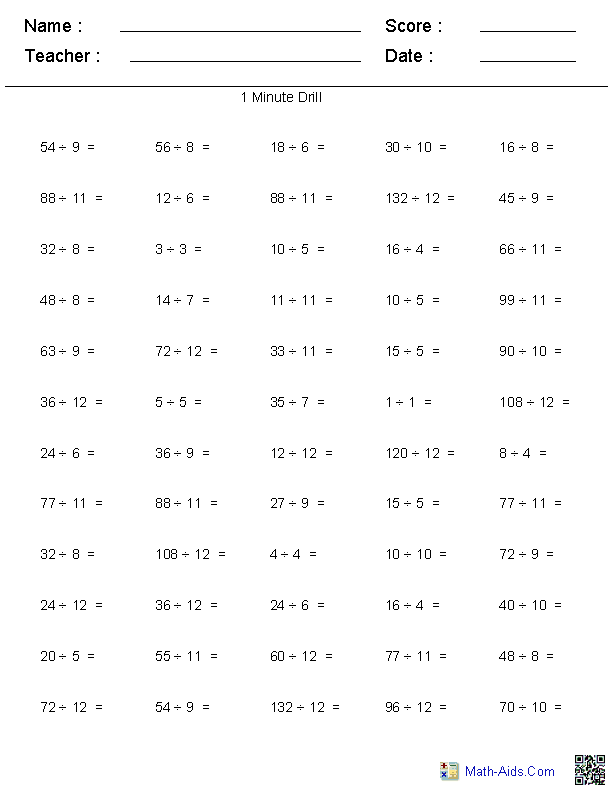##### Division Times TablesTimed Drills Worksheets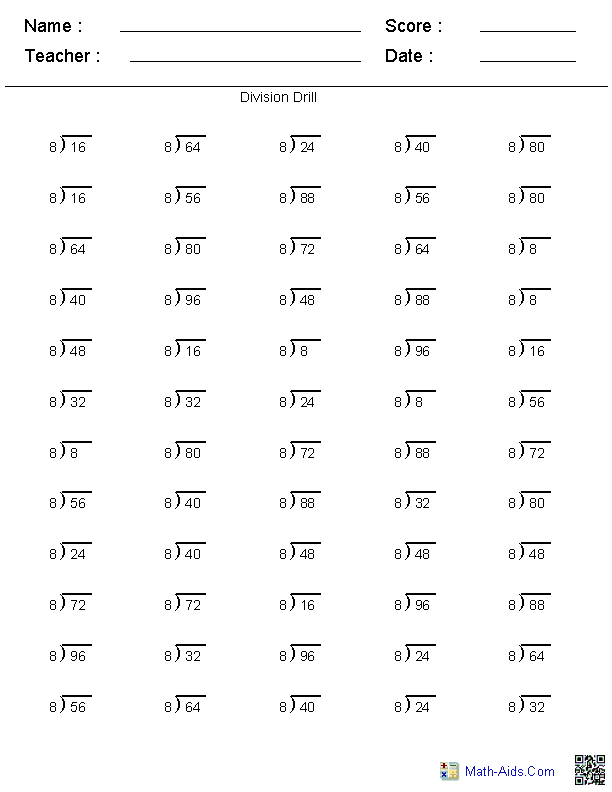##### Missing NumberDivision Worksheets##### Negative NumberDivision Worksheets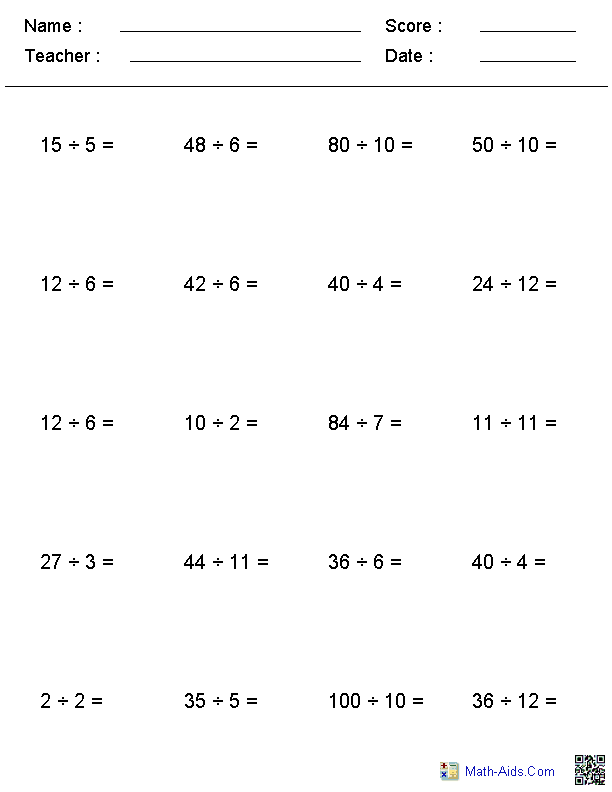##### Writing in Different FormatsDivision Worksheets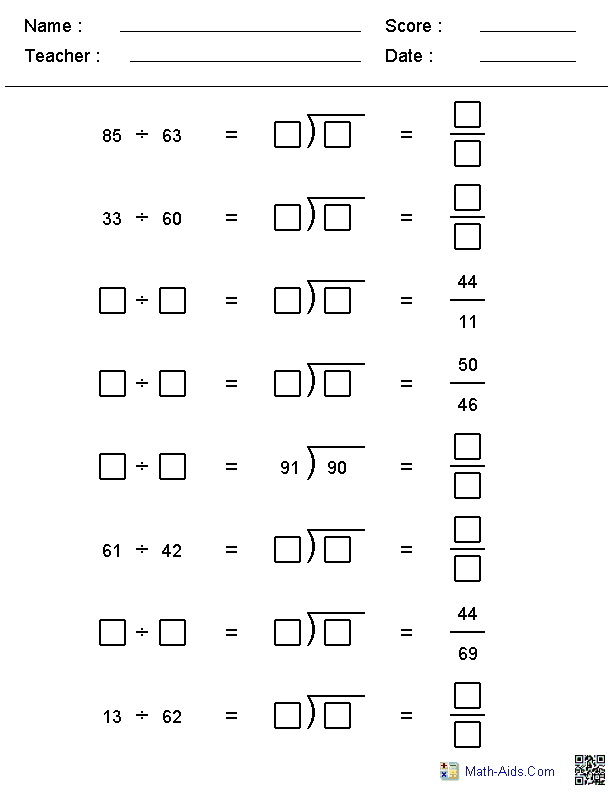##### Horizontal and Long DivisionDivision Worksheets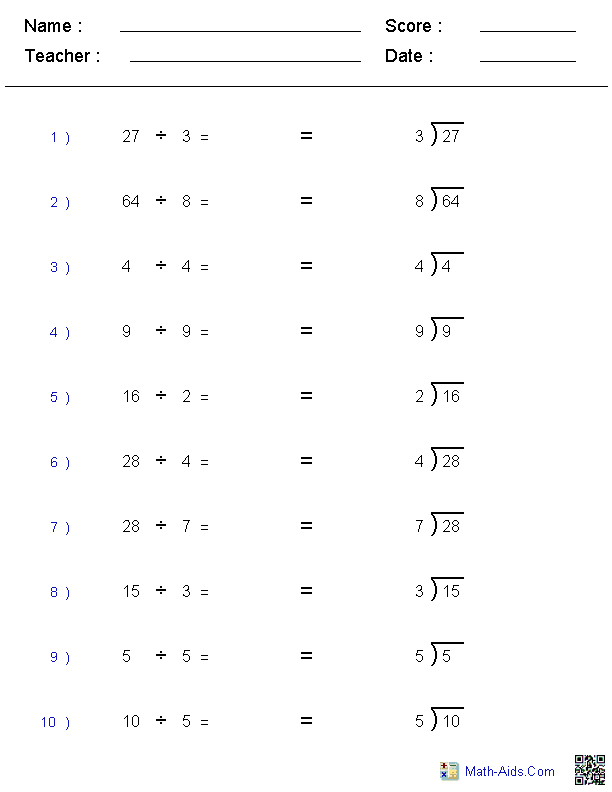##### Division Facts TablesDivision Worksheets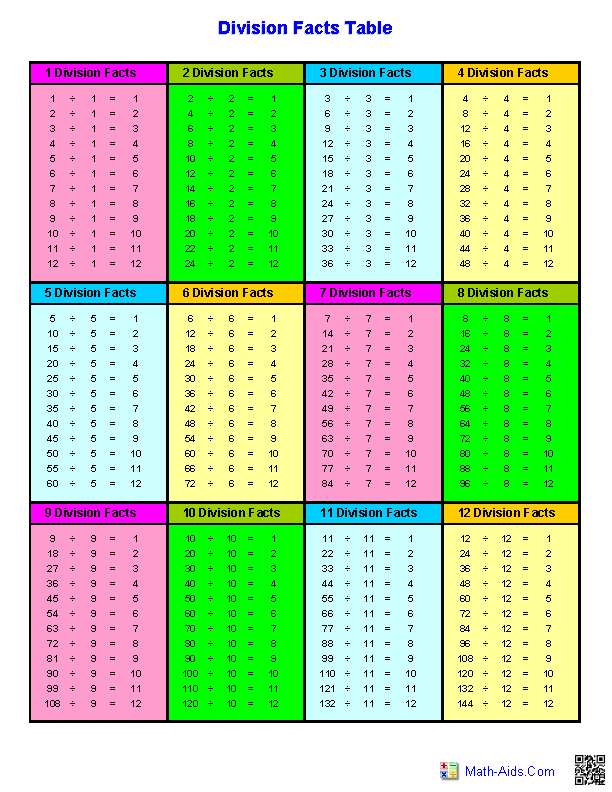Recommended Videos

## Detailed Description for All Division Worksheets

Divisibility Rules Handout Worksheet
This divisibility rules handout worksheet will generate a handout on the rules of divisibility of a number by 2, 3, 4, 5, 6, and 9. This divisibility rules handout worksheet will aid the children in learning the rules.

Divisibility Test Division Worksheets
These division worksheets generate a matrix of numbers for the children to determine if the are divisible by 2, 3, 4, 5, 6, and 9. These division worksheets may be configured with 2, 3, or 4 digit numbers. You may also allow numbers that don't meet any of the divisibility test to increase the difficulty for the children. A divisibility rule worksheet will be created to aid the children in learning the rules.

Divisibility - Pick Divisors Worksheets
This Division Worksheet generates a matrix of numbers for the children to determine if certain numbers are divisible by the divisors you pick. The problems may be configured with 2, 3, or 4 digit numbers. You may also allow pick the divisors to test.

Single or Multiple Digit Division Worksheets
Horizontal Format

These single or multiple digit division worksheets are configured in a horizontal problem format. The numbers for each divisor and quotient may be individually varied to generate different sets of division worksheets. These division worksheets will not have any remainders or fractions. These division worksheets can be configured to layout the problems using the division sign, slash (/), or as a long division format. You may select between 20 and 40 problems for these division worksheets.

Long Division Worksheets
Horizontal Format

These long division worksheets the number of digits for the divisors and quotients may be varied from 1 to 3. You may select whether the long division problems have no remainders, remainders, or mixed. These long division worksheet answer keys may be displayed with a remainder or as a fraction. These long division worksheets may have either 9 or 12 problems per worksheet.

Short Division Worksheets
1 Division - Horizontal Format

These short division worksheets are configured in a horizontal problem format. The numbers for the divisors may range from 2 through 9 for these division worksheets. The number of digits in the quotients may be varied from 1 to 3 digits for these division worksheets. You may also select how may digits will be in the dividend as well. The short division problems may be configured with no remainders, all remainders or mixed remainders. The answer key can be displayed with a remainder or as a fraction. You may select between 20 or 24 problems for these division worksheets.

Decimal Long Division Worksheets
Horizontal Format

These decimal division worksheets allows for the number of digits in the divisors to be varied from 1 to 3. You may select the number of decimals in the dividend for the problems. These decimal division worksheets produce 9 problems per worksheet.

Decimal Divisor Division Worksheets
Horizontal Format

These decimal division worksheet the number of digits for the divisors may be varied between 2 and 3 and the number of decimals in the divisor may be varied between 1 and 2. The decimal divisor division worksheet produces 9 problems per worksheet.

3 Digit Decimal Division Worksheets
Horizontal Format

These Division Worksheets produces problems in which you must divide a 3 digit decimal number by a single digit number. You may select between 12, 15, 18, 21, 24 or 30 problems for these division worksheets.

Mixed Quotient Division Worksheets
These division worksheets will produce problems with mixed formats for the quotient, but keeping the divisor and dividend as whole numbers. You may select either whole numbers, one decimal, two decimals, or a mixture of all types of problems. The division worksheet will produce 9 problems per worksheet.

Division Drills Worksheets

These division drills worksheets contain all of the single digit division problems for one operation on one page. A student should be able to work out the division problems on these division worksheets correctly in the allowed time.

Division Times Tables Timed Drills Worksheets
These division worksheets are for testing the students knowledge of the times tables, but from a division standpoint. The selected times tables will be used as the divisors and quotients in the problems. A student should be able to work out the 60 problems correctly in 3 minutes. You may select which times table to use.

Missing Number Division Worksheets
Horizontal Format

These division worksheets are a good introduction for algebra concepts. You may select various types of characters to replace the missing numbers of the division problems on the division worksheets. The format of the division worksheets are horizontal and the answers range from 0 to 99. These division worksheets can be configured to layout the division problems using the division sign or a slash (/) format. You may select between 12 and 30 problems for these division worksheets.

Negative Number Division Worksheets
Horizontal Format

These division worksheets may be configured for the divisors and quotients in the range of -12 to +12. The format of the division worksheets are horizontal. You may select between 20 and 40 problems for these division worksheets.

Writing Division Problems in Different Formats Division Worksheets
These division worksheets will produce problem sets to teach kids the three common division formats. These division worksheets will generate 8 problems in the long division, horizontal, and fraction format.

Horizontal and Long Division Worksheets
These division worksheets will producing problem sets with horizontal and long division types of formats. The numbers for the divisors may range from 2 through 9. The number of digits in the quotients may be varied from 1 or 2 digits. You may also select how may digits will be in the dividend as well. You may select between 10 or 12 problems for each division worksheets.

Division Facts Tables Worksheet
This division facts table charts are colorful and a great resource for teaching kids their division facts tables. A complete set of printable division facts tables for 1 to 12.